Maths-
General
Easy

Question

# If the tangents drawn from a point on the hyperbola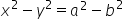to the ellipse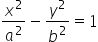make angles α and β with the transverse axis of the hyperbola, then

##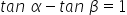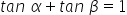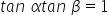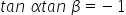Hint:

## The correct answer is:### A hyperbola is a significant conic section in mathematics that is created by the intersection of a double cone with a plane surface, though not always at the centre. A hyperbola is symmetric along its conjugate axis and resembles the ellipse in many ways. A hyperbola is subject to concepts like foci, directrix, latus rectus, and eccentricity.We have given: the tangents drawn from a point on the hyperbolato the ellipsemake angles α and β with the transverse axis of the hyperbola.So here we understood the concept of hyperbola and the normal lines.In analytic geometry, a hyperbola is a conic section created when a plane meets a double right circular cone at an angle that overlaps both cone halves. So the correct relation is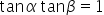#### With Turito Foundation.#### Get an Expert Advice From Turito.